Courses

# NCERT Solutions(Part- 1)- Rational Numbers Class 8 Notes | EduRev

## Class 8 Mathematics by VP Classes

Created by: Vp Classes

## Class 8 : NCERT Solutions(Part- 1)- Rational Numbers Class 8 Notes | EduRev

The document NCERT Solutions(Part- 1)- Rational Numbers Class 8 Notes | EduRev is a part of the Class 8 Course Class 8 Mathematics by VP Classes.
All you need of Class 8 at this link: Class 8

TRY THESE

Ques 1: Fill in the blanks in the following table:

 Numbers Closed under Addition Subtraction Rational numbers Yes Yes Integers ..... Yes Whole numbers ..... ..... Natural numbers ..... No

 Numbers Closed under Multiplication Division Rational numbers .... No Integers ..... No Whole numbers Yes ..... Natural numbers ..... .....

Ans: Using the closure property over addition, subtraction, multiplication, and division for rational numbers, integers, whole-numbers, and natural numbers, we have:

 Numbers Closed under Addition Subtraction Rational numbers Yes Yes Integers Yes Yes Whole numbers Yes No Natural numbers Yes No

 Numbers Closed under Multiplication Division Rational numbers Yes No Integers Yes No Whole numbers Yes No Natural numbers Yes No

Ques 2: Complete the following table:

 Numbers Commutative for Addition Subtraction Rational numbers Yes ..... Integers ..... No Whole numbers ..... ..... Natural numbers ..... .....

 Numbers Commutative for Multiplication Division Rational numbers ..... ..... Integers ..... ..... Whole numbers Yes ..... Natural numbers ..... No

Ans:

 Numbers Commutative for Addition Subtraction Rational numbers Yes No Integers Yes No Whole numbers Yes No Natural numbers Yes No

 Numbers Commutative for Multiplication Division Rational numbers Yes No Integers Yes Yes Whole numbers Yes Yes Natural numbers Yes Yes

Ques 3: Complete the following table:

 Numbers Associative for Addition Subtraction Rational numbers .... .... Integers .... .... Whole numbers Yes .... Natural numbers .... No

 Numbers Associative for Multiplication Division Rational numbers .... No Integers .... .... Whole numbers Yes .... Natural numbers .... ....

Ans:

 Numbers Associative for Addition Subtraction Rational numbers Yes No Integers Yes No Whole numbers Yes No Natural numbers Yes No

 Numbers Associative for Multiplication Division Rational numbers Yes No Integers Yes No Whole numbers Yes No Natural numbers Yes No

Ques 4: If a property holds for rational numbers, will it also hold for integers? For whole Numbers? Which will? Which will not?
Ans:
(i) Any property which is true for rational numbers is also true for integers except for any integers ‘a’ and ‘b’, (a ÷ b) is not necessarily an integer.
(ii) All properties which are true for rational numbers are also true for whole numbers also except:
(a) For ‘a’ and ‘b’ being whole numbers, (a – b) may not be a whole number.
(b) For ‘a’ and ‘b’ being whole numbers (b ≠ 0), a ÷ b may not be the whole number.
Ques 5: Find using distributive property: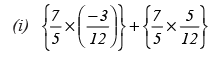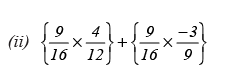Ans: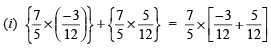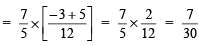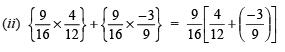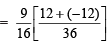(∵ LCM of 12 and 9 is 36)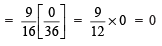EXERCISE 1.1
Ques 1.
Using appropriate properties find: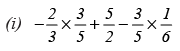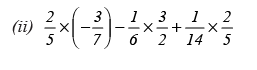Ans: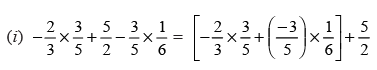(Using commutative property)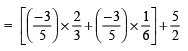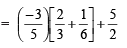(Using distributive property)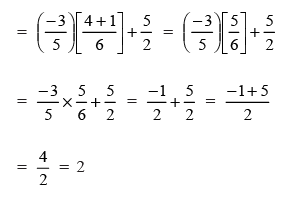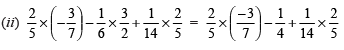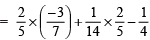(Using commutative property)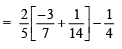(Using distributive property)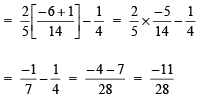Ques 2. Write the additive inverse of each of the following:

(i) 2/8
(ii) -5/9
(iii) -6/-5
(iv) 2/-9
(v) 19/-6

Ans. Concept: The negative of a rational number is called additive inverse.

 Sr. No. Rational number Additive inverse (i)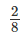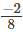(ii)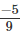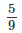(iii) -6/-5 = 6/5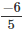(iv)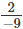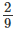(v)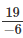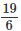Ques 3. Verify that –(–x) = x for: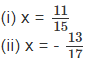Ans.
(i) Given, x = 11/15
⇒ (-x) = -11/15

⇒ -(-x) = -(-11/15) = 11/15
Therefore, -(-x) = x

(ii) Given, x = -13/17
⇒ (-x) = -13/17

⇒ -(-x) = -(-13/17) = 13/17
Therefore, -(-x) = x

Ques 4. Find the multiplicative inverse of the following: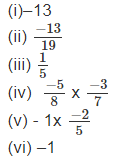Ans:

 Sr. No. Rational number Multiplicative inverse (i) –13 -1/13 (ii) -13/19 -19/13 (iii) 1/5 5 (iv)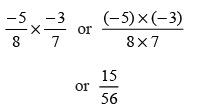56/15 (v)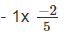5/2 (vi) -1 -1

Ques 5: Name the property under multiplication used in each of the following: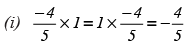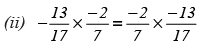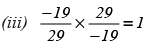Ans:

 Sr. No. Multiplication Property used (i)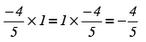1 is the multiplicative identity (ii)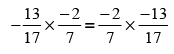Commutative property (iii)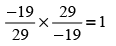Multiplicative inverse

Ques 6: Multiply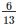by the reciprocal of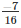Ans: As,
Reciprocal of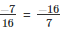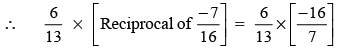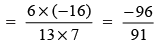Ques 7: Tell what property allows you to compute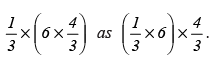Ans: When rational numbers are rearranged between one or more same operations and still their result does not change then we say that they follow the associative property for that operation. Thus, given equation follows the associative property.

Ques 8: Is  8/9 the multiplicative inverse of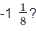Why or why not?
Ans: Since,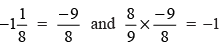[Which is not equal to 1]

∴ 8/9 is not the multiplicative inverse of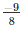Ques 9: Is 0.3 the multiplicative inverse of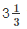? Why or why not?
Ans: Since,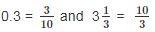0.3 х 10/3 = 1

Therefore, 0.3 is the multiplicative inverse of 10/3.

Ques 10: Write:
(i) The rational number that does not have reciprocal.
(ii) The rational numbers that are equal to their reciprocals.
(iii) The rational number that is equal to its negative.
Ans:
(i) The rational number zero (0) does not have a reciprocal.
(ii) The rational numbers 1 and (–1) are equal to their reciprocals respectively.
(iii) Zero (0) is the rational number that is equal to its negative.

Ques 11: Fill in the blanks:
(i) Zero has ______ reciprocal.
(ii) The numbers ______ and ______ are their own reciprocals.
(iii) The reciprocal of –5 is ______.
(iv) Reciprocal of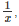, where x ≠ 0 is ______.
(v) The product of two rational numbers is always a ______.
(vi) The reciprocal of a positive rational number is ______.

Ans:
(i) Zero has no reciprocal.

(ii) The numbers 1 and –1 are their own reciprocals.
(iii) The reciprocal of –5 is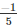(iv) The reciprocal of  1/x , where x ≠ 0 is x.
(v) The product of two rational numbers is always a rational number.
(vi) The reciprocal of a positive rational number is positive.

Offer running on EduRev: Apply code STAYHOME200 to get INR 200 off on our premium plan EduRev Infinity!

93 docs|16 tests

,

,

,

,

,

,

,

,

,

,

,

,

,

,

,

,

,

,

,

,

,

;